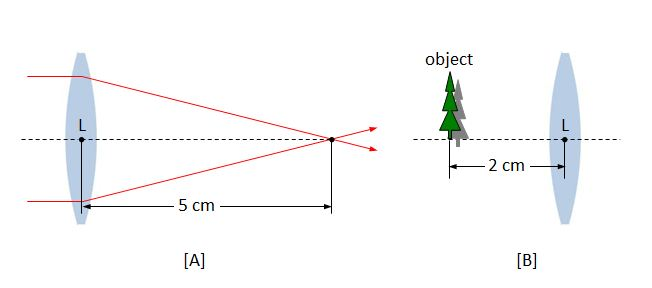# Thin lens formulaDiagram [A] above shows a biconvex lens through which a collimated beam travelling along the lens axis is focused on a spot (known as the focal point) at a distance of $5\text{ cm}$ from L, the center of the lens. If we locate an object $2\text{ cm}$ to the left of L as in diagram [B], then where will be the position of the image of the object?

×

Problem Loading...

Note Loading...

Set Loading...